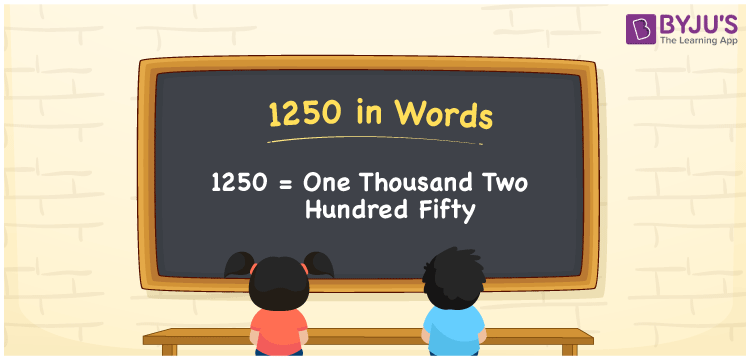# 1250 in Words

1250 in words can be written as One Thousand Two Hundred Fifty. You will learn in detail regarding the concept of counting which is essential in our daily lives. If you buy a raincoat for Rs. 1250, then you can say that “I bought a raincoat for One Thousand Two Hundred and Fifty Rupees”. Here numbers in words are written using the English alphabet. Therefore, the number 1250 can be read as “One Thousand Two Hundred Fifty” in words.

 1250 in words One Thousand Two Hundred Fifty One Thousand Two Hundred Fifty in Numbers 1250

## 1250 in English Words## How to Write 1250 in Words?

Learn in detail about the place value chart of 1250 below. Four digits are present in 1250 and the place value of each can be understood from the table given here.

 Thousands Hundreds Tens Ones 1 2 5 0

1250 in expanded form is:

1 x Thousand + 2 × Hundred + 5 × Ten + 0 × One

= 1 x 1000 + 2 × 100 + 5 × 10 + 0 × 1

= 1000 + 200 + 50

= 1250

= One Thousand Two Hundred and Fifty

Hence, 1250 in words is written as One Thousand Two Hundred Fifty.

1250 is a natural number that precedes 1251 and succeeds 1249.

1250 in words – One Thousand Two Hundred Fifty

Is 1250 an odd number? – No

Is 1250 an even number? – Yes

Is 1250 a perfect square number? – No

Is 1250 a perfect cube number? – No

Is 1250 a prime number? – No

Is 1250 a composite number? – Yes

## Frequently Asked Questions on 1250 in Words

### How can 1250 be written in words?

1250 can be written as “One Thousand Two Hundred Fifty” in words.

### What is 1500 – 250 in words?

1500 – 250 is 1250. It can be written as One Thousand Two Hundred Fifty in words.

### Write One Thousand Two Hundred Fifty in numbers.

One Thousand Two Hundred Fifty can be written in numbers as 1250.